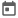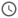# Conditional Statements in Verilog##### 16 Sep 2021##### 4 mins

Conditional statements in a programming language help to branch the execution of code depending on some condition. In simpler words, using these statements, the execution of specific code lines can be controlled using some conditions. Verilog provides two types of conditional statements, namely

1. If-else
2. Case statement

Let us explore both statements in detail.

### If-else

If-else is the most straightforward conditional statement construct. For the compiler, this statement means that “If some condition is true, execute code inside if or else execute codes inside else.” The condition evaluates to be true if the argument is a non-zero number. In if-else, it is not necessary to always have an else block.

#### Syntax:

``````if(<condition>) begin
<code>
end
else begin
<code>
end``````

### If-else-if

If-else-if construct is used when more than one condition needs to be checked. All the conditions are checked serially, and if none of the conditions is true, then the `else` block is executed.

#### Syntax:

``````if(<condition>) begin
<code>
end
else if(<condition>) begin
<code>
end
else begin
<code>
end``````

### Case Statements

Case statements are generally used when the condition is an equivalence check, which means “==” or “===”. Case statement makes the code more readable wrt to if-else if there are many conditions to check. As the name indicates, this statement will switch a particular case based on some arguments.

#### Syntax:

``````case(<argument>)
<case-1>: <single-line code>
<case-2>: begin
<code>
end
default: <code>
endcase``````

Again, to account for the four-state variables, there are three case statement variants: `case`, `casez`, and `casex`. Let us look at them in detail and also find out the difference.

#### Case

In the case statement, exact matching is done. If even a single bit does not match the cases defined, the default case will be executed.

``````module case_demo;
reg [1:0] op_code;
initial begin
op_code = 2'd1;
\$display("Op code is %0d", op_code);
#10;
op_code = 2'd2;
\$display("Op code is %0d", op_code);
#10;
op_code = 2'bx;
\$display("Op code is %0d", op_code);
#10;
op_code = 2'bz1;
\$display("Op code is %0d", op_code);
end

always @(op_code) begin
case(op_code)
2'b00: begin
\$display("Case 0 selected");
end
2'b01: begin
\$display("Case 1 selected");
end
2'bz1: begin
\$display("Case z1 selected");
end
default: begin
\$display("No operation for this case");
end
endcase
end
endmodule``````
##### Output
``````# Op code is 1
# Case 1 selected
# Op code is 2
# No operation for this case
# Op code is x
# No operation for this case
# Op code is Z
# Case z1 selected``````

#### Casez

In the previous statement, we have seen that a case will be executed if it matches precisely with the argument. However, in many cases, we would not want to match the cases exactly. Consider a simple example where the master uses a select signal to select a particular slave amongst many (say, four slaves). Thus, the select line would be a 4-bit signal. A single bit needs to be high to select any slave, and the rest of the bits can be anything. Thus, instead of writing multiple cases for a slave, we can use casez. In casez, the z or ‘?’ is does not care, which means it will not be considered while comparing the case with the argument.

##### Example
``````module casez_demo;
reg [1:0] op_code;

initial begin
op_code = 2'd1;
\$display("Op code is %0d", op_code);
#10;
op_code = 2'd2;
\$display("Op code is %0b", op_code);
#10;
op_code = 2'b1z;
\$display("Op code is %0b", op_code);
#10;
op_code = 2'b11;
\$display("Op code is %0b", op_code);
#10;
op_code = 2'b0z;
\$display("Op code is %0b", op_code);
end

always @(op_code) begin
casez(op_code)
// 2'b01: begin
//     \$display("Case **01** selected");
// end
2'b00: begin
\$display("Case **00** selected");
end
2'b01: begin
\$display("Case **01** selected");
end
2'b1?: begin
\$display("Case **1?** selected");
end
default: begin
\$display("No operation for this case");
end
endcase
end
endmodule``````
##### Output
``````# Op code is 1
# Case **01** selected
# Op code is 10
# Case **1?** selected
# Op code is 1z
# Case **1?** selected
# Op code is 11
# Case **1?** selected
# Op code is 0z
# Case **00** selected``````

#### Casex

Casex is a bit more flexible than casez. In casex, even `X` is not considered during case comparison. Thus, `X`, `Z`, `?` all are considered as do not care in casex. Let us see the difference between all these case statements using an example.

##### Example

In the below example, the same operations are performed in different always block but using a different case statement. In the output, it can be seen that, if the value of the argument, i.e., `op_code` is `X`, then default case is executed in the `case` and `casez` construct, but for `casex`, the `X` is considered as do not care, and thus the first case is executed.
When the `op_code` is `Z`, then only in the case construct the default case is executed, and for the `casex` and `casez`, Z is considered as do not care, and the 1st case is executed.

``````module case_diff_demo;
reg [7:0] a, b, op, op_x, op_z;
reg [1:0] op_code;

always @(a or b or op_code) begin
case(op_code)
2'b00: begin
op = a + b;
end
2'b01: begin
op = a - b;
end
default
op = 8'd0;
endcase
\$display("Output of case = %0d", op);
end

always @(a or b or op_code) begin
casez(op_code)
2'b00: begin
op_z = a + b;
end
2'b01: begin
op_z = a - b;
end
default
op_z = 8'd0;
endcase
\$display("Output of casez = %0d", op_z);
end

always @(a or b or op_code) begin
casex(op_code)
2'b00: begin
op_x = a + b;
end
2'b01: begin
op_x = a - b;
end
default
op_x = 8'd0;
endcase
\$display("Output of casex = %0d", op_x);
end

initial begin
a = 8'd12;
b = 8'd4;
op_code = 0;
\$display("op_code = %0d", op_code);
#10;
op_code = 1;
\$display("op_code = %0d", op_code);
#10;
op_code = 3;
\$display("op_code = %0d", op_code);
#10;
op_code = 2'bx;
\$display("op_code = %0d", op_code);
#10;
op_code = 2'bz;
\$display("op_code = %0d", op_code);
end
endmodule``````
##### Output
``````# op_code = 0
# Output of case = 16
# Output of casez = 16
# Output of casex = 16
# op_code = 1
# Output of case = 8
# Output of casez = 8
# Output of casex = 8
# op_code = 3
# Output of case = 0
# Output of casez = 0
# Output of casex = 0
# op_code = x
# Output of case = 0
# Output of casez = 0
# Output of casex = 16
# op_code = z
# Output of case = 0
# Output of casez = 16
# Output of casex = 16``````
Try this code in EDA Playground

All three case statements are synthesizable, but it is not recommended to use casex and casez in designs. It is because the output after synthesis and during the simulation can differ in casex and casez. Case statements come in behavioural modelling (we will learn more about in future topics), and for complex behavioural logics, the synthesis can be different in different tools.